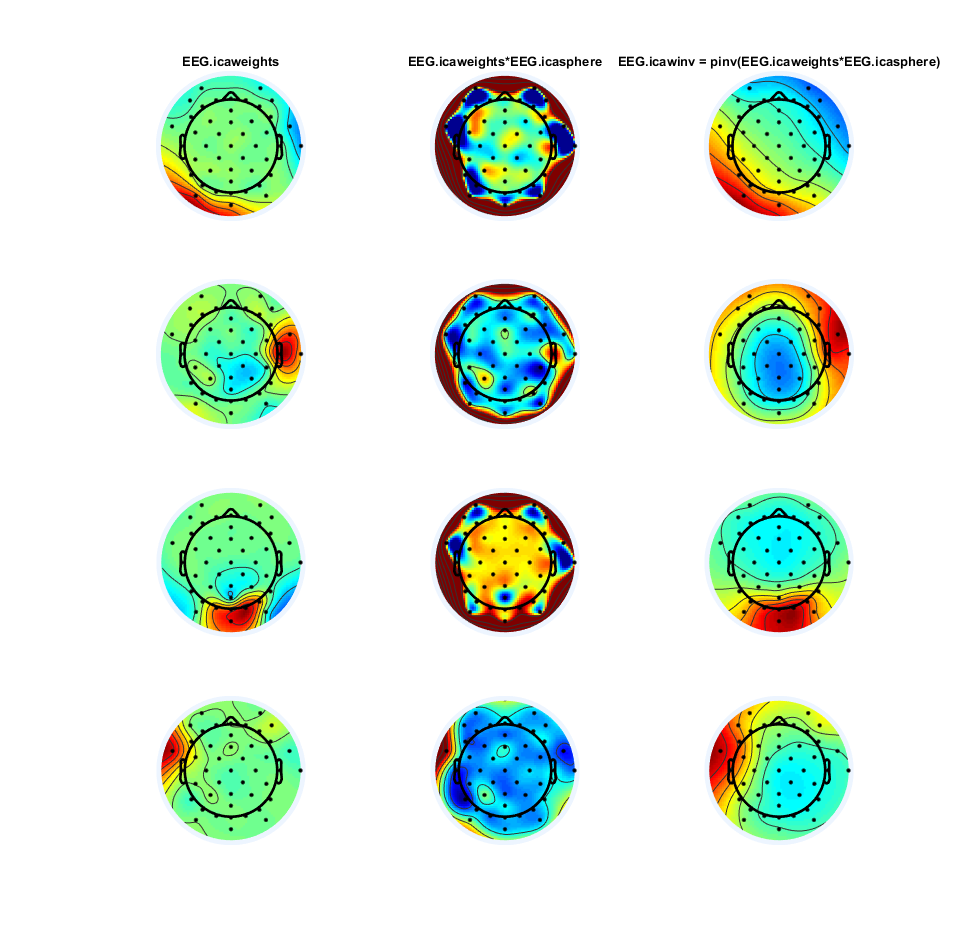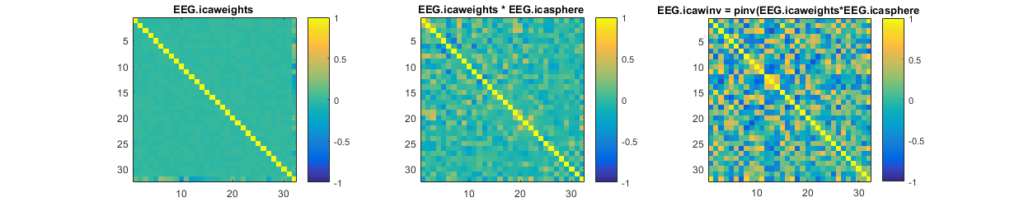# ICA weights and invweights

## Update 01.08.2016

after further discussion we discovered that the correlation matrix was a bit off. This is because I plotted the correlation of the weights without the sphering (=whitening) step. Sphering removes the correlation between the components. I added a plot with the correlation after sphering and updated the topographies.

## Goal

In EEG blind source separation using ICA, understand the difference between the mixing matrix and the unmixing matrix.

## brief introduction

The blind source separation problem in the context of EEG can be understood as follows:
$$mixing * sourceactivity = EEGdata$$
In words: We have some sources active and we know their projections on each electrode: We take the dot product (we multiply each source with its projection and add them all up).
More formally:

\begin{align} A &: mixing\\ s &: sourceactivity\\ x &: EEGdata \\ A * s &= x\\ \end{align}
Because every neuronal source projects to most (or even all) sensors/electrodes, the original brain-source activation can only be recored in a mixed way.
Thus in order to unmix we can use blind source separation (ICA details can be found here)
\begin{align} W &: unmixing \\ unmixing * EEG.data &= sourceactivity \\ W * x &= s \\ \end{align}
The relation between mixing and unmixing matrix in ICA (and other BSS methods) is:

\begin{align} A * s & = x\\ A^{-1} * A * s & = A^{-1} * x\\ s & = A^{-1} * x\\ A^{-1}& = W\\ \end{align}
This is only true if $A$ is invertible and square (if non-square you have to use a pseudoinverse, see the Haufe 2014 paper for more information)

The matrix $W$ is also known as the extraction filter

The matrix $A$ is also know as the activity pattern

## EEG ICAs

In eeglab the default infomax-ICA algorithm uses a sphering (whitening) step before calculating the weights. Thus the following relation is true:

[code lang=text] EEG.icawinv = pinv( EEG.icaweights * EEG.icasphere) % the mixing matrix, channels x sources
EEG.icaact = EEG.icaweights*EEG.icasphere)*EEG.data % the activation, sources x time
[/code]

This is how ICA components look, on the left the weights after sphering-preprocessing, middle the unmixing matrix and on the right the mixing matrix. Usually we should look at the mixing matrix ($A = W^{-1}$).The weights after decorrelation (=sphering) seem to be quite similar, but the unmixing ($W$) looks very different and is actually very hard to interprete!
One interesting thing to look at is the correlation between the component-vectors (in this case 32 components). As we can see here:Note that the correlation of the EEG.icaweight matrix is 0 because it is a pure rotation matrix (which is orthognal, thus correlations of 0). As explained here the whitening step estimates the stretching and thus only a rotation remains for the EEG.icaweight matrix.

Haufe et. al. 2014(pdf-openaccess-link) have a very nice paper around this issue.

TLDR; Don’t look at your filter/unmixing matrix.

### Thanks to

Holger Finger, Anna Lisa Gert, Tim Kietzmann,Peter König, Andrew Melnik ( in alphabetical order) for discussions.

01-2023: Thanks to Fabiano Baroni for noticing I had $$W$$ & $$A$$ switched up throughout the blog post!!

Categorized: Blog

Tagged:

1.•